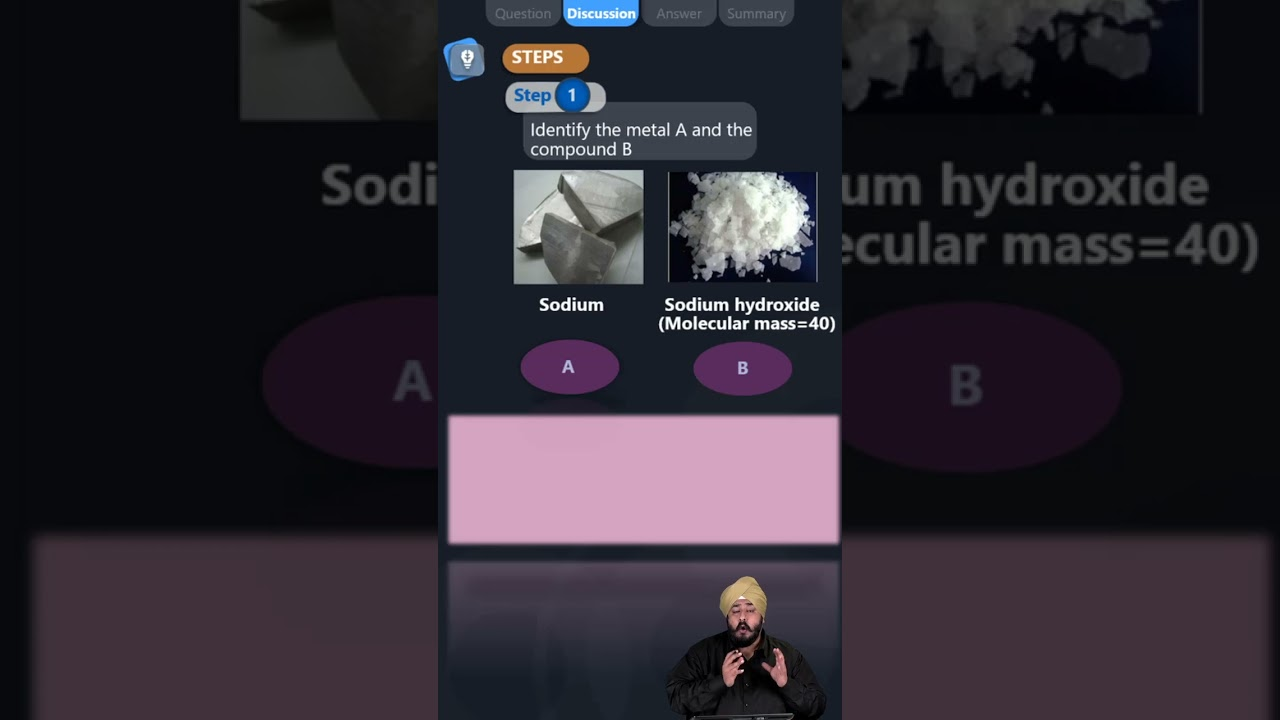# An alkali metal A gives a compound B (molecular mass = 40) on reacting with water. The compound B gives a soluble compound C on treatment with aluminium oxide. Identify A, B and C and give the reaction involved.According to the question,

Given

• x + 16 + 1 = 40
• x = 40 – 17 = 23

It is the atomic weight of Na (sodium).

Therefore, the alkali metal (A) is Na and the reaction is

2Na + 2H2O → 2NaOH + H2

So, compound B is sodium hydroxide (NaOH).

Sodium hydroxide reacts with aluminium oxide (Al2O3) to give sodium aluminate (NaAlO2). Thus, C is sodium aluminate (NaAlO2). The reaction involved is

Al2O3 + 2NaOH → 2NaAlO2 + H2O

Hence

• A is sodium
• B is Sodium Hydroxide
• C is Sodium Aluminate(8)(1)